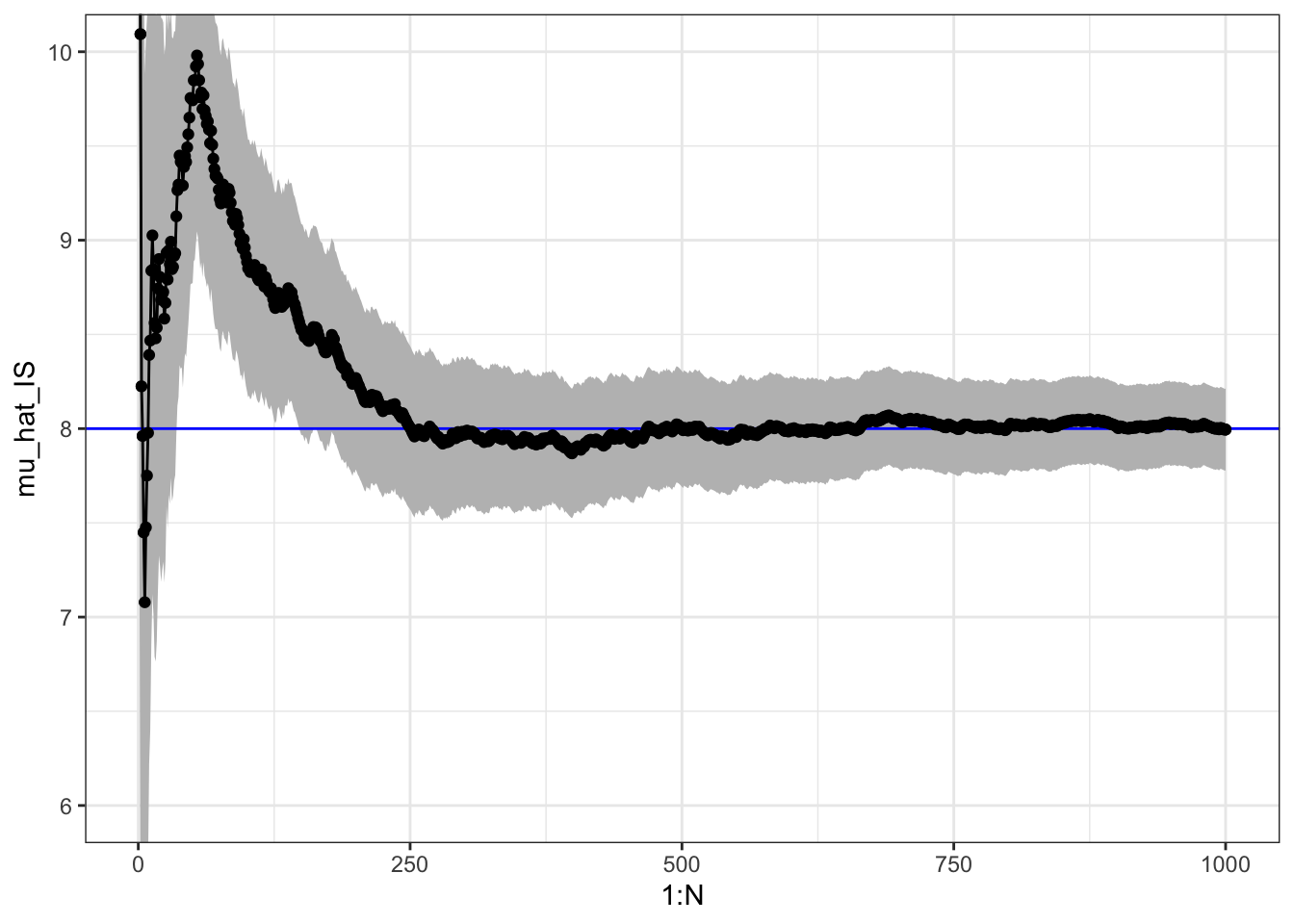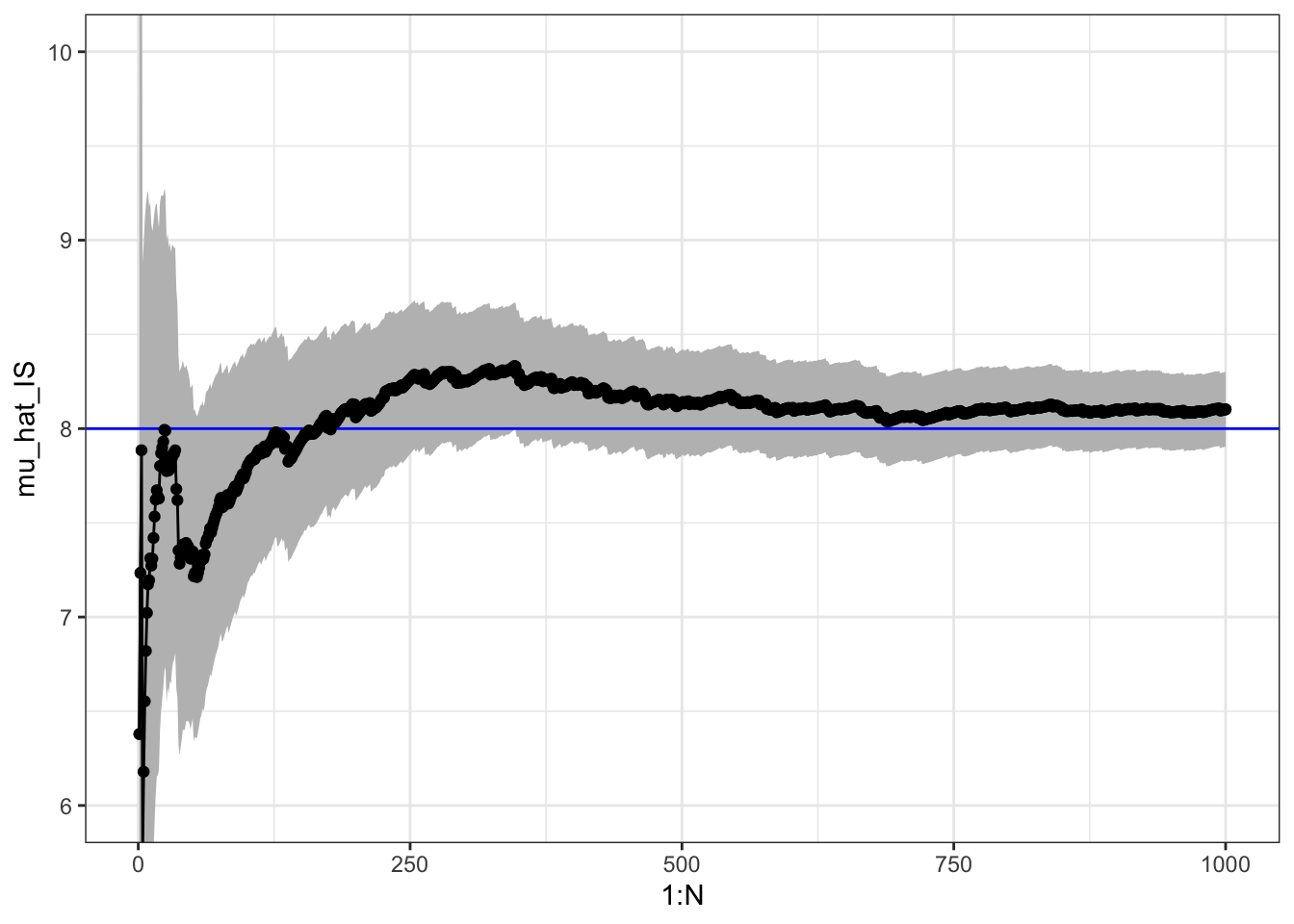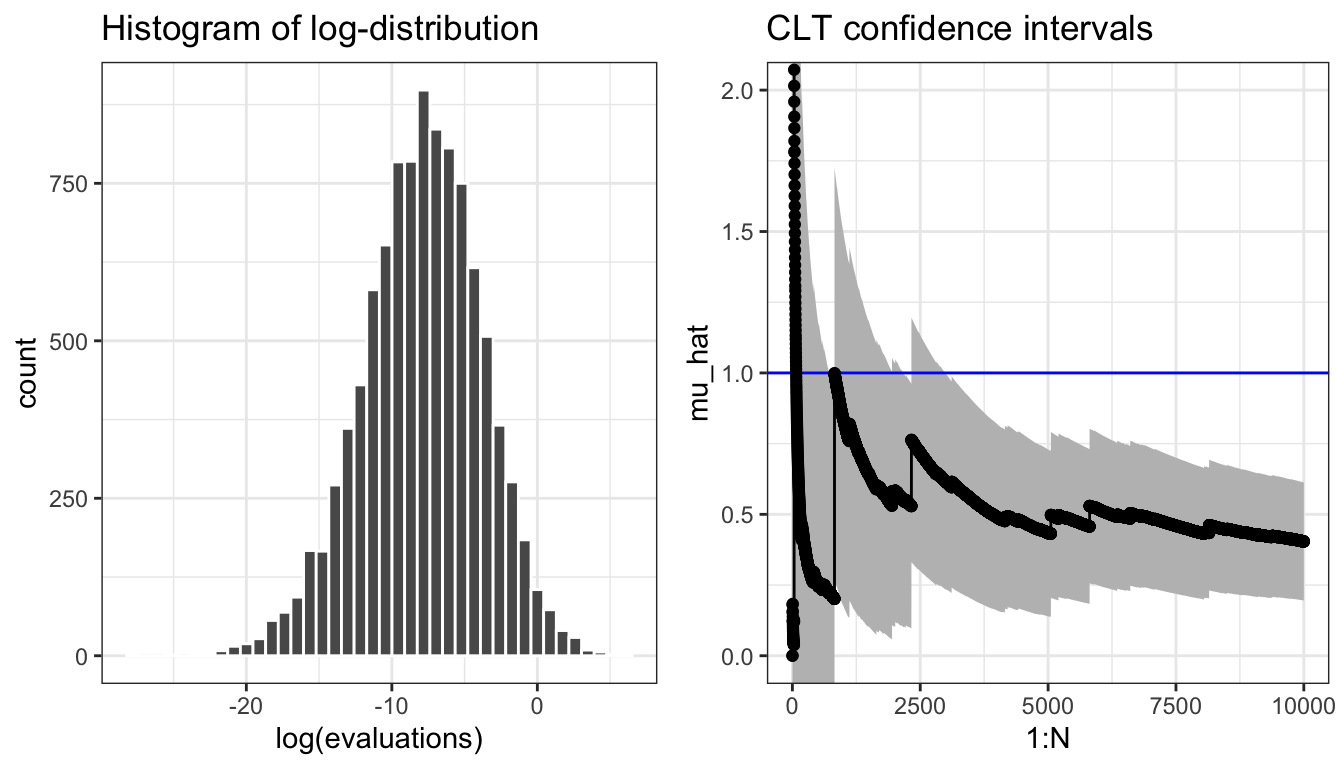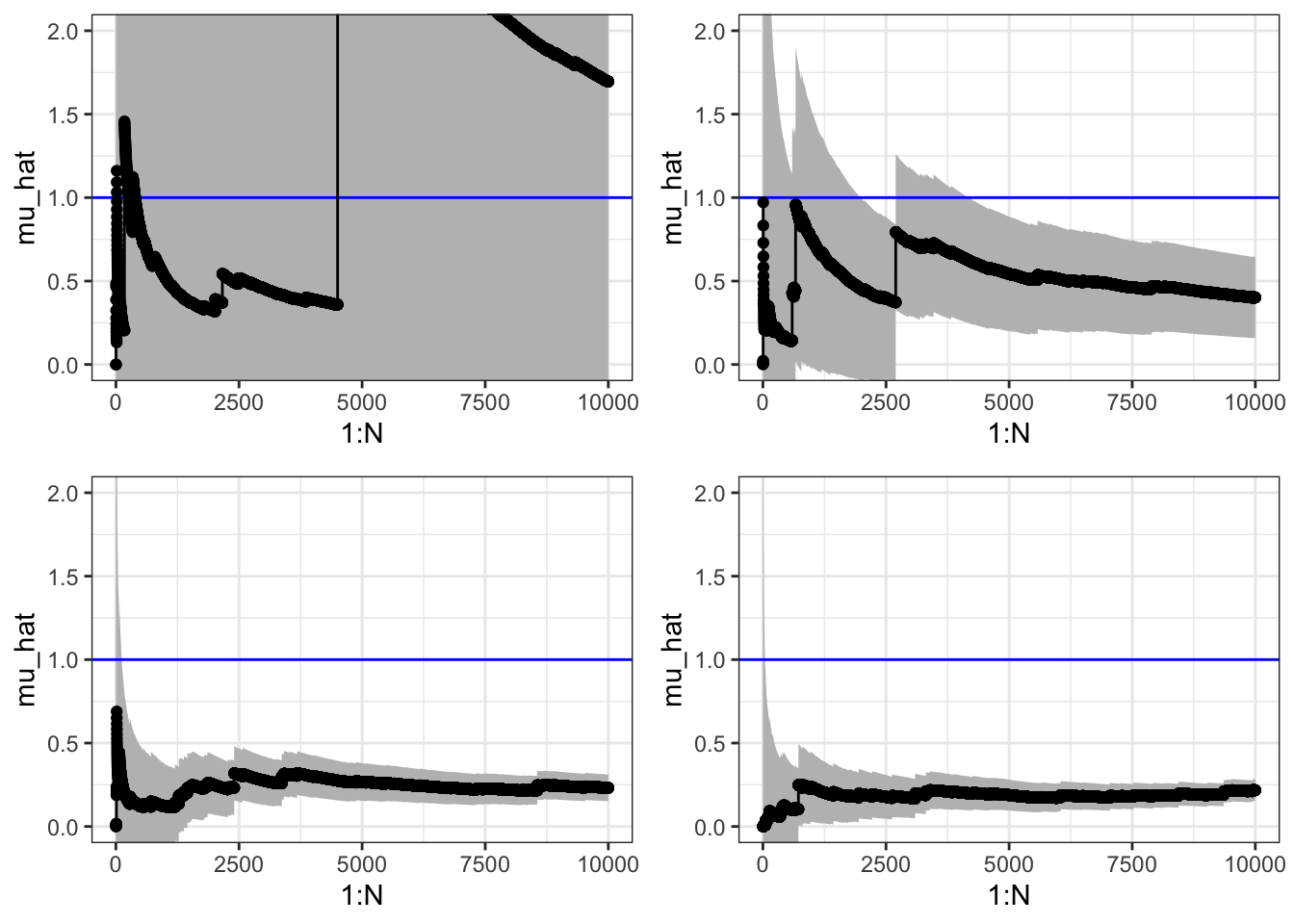## 5.2 Importance sampling

When we are only interested in Monte Carlo integration, we do not need to sample from the target distribution.

Observe that \begin{align} \mu = \int h(x) f(x) \ \mathrm{d}x & = \int h(x) \frac{f(x)}{g(x)} g(x) \ \mathrm{d}x \\ & = \int h(x) w^*(x) g(x) \ \mathrm{d}x \end{align}

whenever $$g$$ is a density fulfilling that $g(x) = 0 \Rightarrow f(x) = 0.$

With $$X_1, \ldots, X_n$$ i.i.d. with density $$g$$ define the weights $w^*(X_i) = f(X_i) / g(X_i).$ The importance sampling estimator is $\hat{\mu}_{\textrm{IS}}^* := \frac{1}{n} \sum_{i=1}^n h(X_i)w^*(X_i).$ It has mean $$\mu$$. Again by the LLN $\hat{\mu}_{\textrm{IS}}^* \rightarrow E(h(X_1) w^*(X_1)) = \mu.$

We will illustrate the use of importance sampling by computing the mean in the gamma distribution via simulations from a Gaussian distribution, cf. also Section 5.1.1.

x <- rnorm(n, 10, 3)
w_star <- dgamma(x, 8) / dnorm(x, 10, 3)
mu_hat_IS <- (cumsum(x * w_star) / (1:n))
mu_hat_IS[n]  # Theoretical value 8
##  7.995228

To assess the precision of the importance sampling estimate via the CLT we need the variance of the average just as for plain Monte Carlo integration. By the CLT $\hat{\mu}_{\textrm{IS}}^* \overset{\textrm{approx}} \sim \mathcal{N}(\mu, \sigma^{*2}_{\textrm{IS}} / n)$ where $\sigma^{*2}_{\textrm{IS}} = V (h(X_1)w^*(X_1)) = \int (h(x) w^*(x) - \mu)^2 g(x) \ \mathrm{d}x.$

The importance sampling variance can be estimated similarly as the Monte Carlo variance $\hat{\sigma}^{*2}_{\textrm{IS}} = \frac{1}{n - 1} \sum_{i=1}^n (h(X_i)w^*(X_i) - \hat{\mu}_{\textrm{IS}}^*)^2,$ and a 95% standard confidence interval is computed as $\hat{\mu}^*_{\textrm{IS}} \pm 1.96 \frac{\hat{\sigma}^*_{\textrm{IS}}}{\sqrt{n}}.$

sigma_hat_IS <- sd(x * w_star)
sigma_hat_IS  # Theoretical value ??
##  3.499995Figure 5.3: Sample path with confidence band for importance sampling Monte Carlo integration of the mean of a gamma distributed random variable via simulations from a Gaussian distribution.

It may happen that $$\sigma^{*2}_{\textrm{IS}} > \sigma^2_{\textrm{MC}}$$ or $$\sigma^{*2}_{\textrm{IS}} < \sigma^2_{\textrm{MC}}$$ depending on $$h$$ and $$g$$, but by choosing $$g$$ cleverly so that $$h(x) w^*(x)$$ becomes as constant as possible, importance sampling can often reduce the variance compared to plain Monte Carlo integration.

For the mean of the gamma distribution above, $$\sigma^{*2}_{\textrm{IS}}$$ is about 50% larger than $$\sigma^2_{\textrm{MC}}$$, so we loose precision by using importance sampling this way when compared to plain Monte Carlo integration. In Section 5.3 we consider a different example where we achieve a considerable variance reduction by using importance sampling.

### 5.2.1 Unknown normalization constants

If $$f = c^{-1} q$$ with $$c$$ unknown then $c = \int q(x) \ \mathrm{d}x = \int \frac{q(x)}{g(x)} g(x) \ d x,$ and $\mu = \frac{\int h(x) w^*(x) g(x) \ d x}{\int w^*(x) g(x) \ d x},$ where $$w^*(x) = q(x) / g(x).$$

If $$X_1, \ldots, X_n$$ are then i.i.d. from the distribution with density $$g$$, an importance sampling estimate of $$\mu$$ can be computed as $\hat{\mu}_{\textrm{IS}} = \frac{\sum_{i=1}^n h(X_i) w^*(X_i)}{\sum_{i=1}^n w^*(X_i)} = \sum_{i=1}^n h(X_i) w(X_i),$ where $$w^*(X_i) = q(X_i) / g(X_i)$$ and $w(X_i) = \frac{w^*(X_i)}{\sum_{i=1}^n w^*(X_i)}$ are the standardized weights. This works irrespectively of the value of the normalizing constant $$c$$, and it actually works if also $$g$$ is unnormalized.

Revisiting the mean of the gamma distribution, we can implement importance sampling via samples from a Gaussian distribution but using weights computed without the normalization constants.

w_star <- numeric(n)
x_pos <- x[x > 0]
w_star[x > 0] <- exp((x_pos - 10)^2 / 18 - x_pos + 7 * log(x_pos))
mu_hat_IS <- cumsum(x * w_star) / cumsum(w_star)
mu_hat_IS[n]  # Theoretical value 8
##  8.102177

The variance of the IS estimator with standardized weights is a little more complicated, because the estimator is a ratio of random variables. From the multivariate CLT $\frac{1}{n} \sum_{i=1}^n \left(\begin{array}{c} h(X_i) w^*(X_i) \\ w^*(X_i) \end{array}\right) \overset{\textrm{approx}}{\sim} \mathcal{N}\left( c \left(\begin{array}{c} \mu \\ {1} \end{array}\right), \frac{1}{n} \left(\begin{array}{cc} \sigma^{*2}_{\textrm{IS}} & \gamma \\ \gamma & \sigma^2_{w^*} \end{array} \right)\right),$ where \begin{align} \sigma^{*2}_{\textrm{IS}} & = V(h(X_1)w^*(X_1)) \\ \gamma & = \mathrm{cov}(h(X_1)w^*(X_1), w^*(X_1)) \\ \sigma_{w^*}^2 & = V (w^*(X_1)). \end{align}

We can then apply the $$\Delta$$-method with $$t(x, y) = x / y$$. Note that $$Dt(x, y) = (1 / y, - x / y^2)$$, whence $Dt(c\mu, c) \left(\begin{array}{cc} \hat{\sigma}^{*2}_{\textrm{IS}} & \gamma \\ \gamma & \sigma^2_{w^*} \end{array} \right) Dt(c\mu, c)^T = c^{-2} (\sigma^{*2}_{\textrm{IS}} + \mu^2 \sigma_{w^*}^2 - 2 \mu \gamma).$

By the $$\Delta$$-method $\hat{\mu}_{\textrm{IS}} \overset{\textrm{approx}}{\sim} \mathcal{N}(\mu, c^{-2} (\sigma^{*2}_{\textrm{IS}} + \mu^2 \sigma_{w^*}^2 - 2 \mu \gamma) / n).$ The unknown quantities in the asymptotic variance must be estimated using e.g.  their empirical equivalents, and if $$c \neq 1$$ (we have used unnormalized densities) it is necessary to estimate $$c$$ as $$\hat{c} = \frac{1}{n} \sum_{i=1}^n w^*(X_i)$$ to compute an estimate of the variance.

For the example with the mean of the gamma distribution, we find the following estimate of the variance.

c_hat <- mean(w_star)
sigma_hat_IS <- sd(x * w_star)
sigma_hat_w_star <- sd(w_star)
gamma_hat <- cov(x * w_star, w_star)
sigma_hat_IS_w <- sqrt(sigma_hat_IS^2 + mu_hat_IS[n]^2 * sigma_hat_w_star^2 -
2 * mu_hat_IS[n] * gamma_hat) / c_hat
sigma_hat_IS_w
##  3.198314Figure 5.4: Sample path with confidence band for importance sampling Monte Carlo integration of the mean of a gamma distributed random variable via simulations from a Gaussian distribution and using standardized weights.

In this example, the variance when using standardized weights is a little lower than using unstandardized weights, but still larger than the variance for the plain Monte Carlo average.

### 5.2.2 Computing a high-dimensional integral

To further illustrate the usage but also the limitations of Monte Carlo integration, consider the following $$p$$-dimensional integral $\int e^{-\frac{1}{2}\left(x_1^2 + \sum_{i=2}^p (x_i - \alpha x_{i-1})^2\right)} \mathrm{d} x.$ Now this integral is not even expressed as an expectation w.r.t. any distribution in the first place – it is an integral w.r.t. Lebesgue measure in $$\mathbb{R}^p$$. We use the same idea as in importance sampling to rewrite the integral as an expectation w.r.t. a probability distribution. There might be many ways to do this, and the following is just one.

Rewrite the exponent as $||x||_2^2 + \sum_{i = 2}^p \alpha^2 x_{i-1}^2 - 2\alpha x_i x_{i-1}$ so that

\begin{align*} \int e^{-\frac{1}{2}\left(x_1^2 + \sum_{i=2}^p (x_i - \alpha x_{i-1})^2\right)} \mathrm{d} x & = \int e^{- \frac{1}{2} \sum_{i = 2}^p \alpha^2 x_{i-1}^2 - 2\alpha x_i x_{i-1}} e^{-\frac{||x||_2^2}{2}} \mathrm{d} x \\ & = (2 \pi)^{p/2} \int e^{- \frac{1}{2} \sum_{i = 2}^p \alpha^2 x_{i-1}^2 - 2\alpha x_i x_{i-1}} f(x) \mathrm{d} x \end{align*}

where $$f$$ is the density for the $$\mathcal{N}(0, I_p)$$ distribution. Thus if $$X \sim \mathcal{N}(0, I_p)$$, $\int e^{-\frac{1}{2}\left(x_1^2 + \sum_{i=2}^p (x_i - \alpha x_{i-1})^2\right)} \mathrm{d} x = (2 \pi)^{p/2} E\left( e^{- \frac{1}{2} \sum_{i = 2}^p \alpha^2 X_{i-1}^2 - 2\alpha X_i X_{i-1}} \right).$

The Monte Carlo integration below computes $\mu = E\left( e^{- \frac{1}{2} \sum_{i = 2}^p \alpha^2 X_{i-1}^2 - 2\alpha X_i X_{i-1}} \right)$ by generating $$p$$-dimensional random variables from $$\mathcal{N}(0, I_p)$$. It can actually be shown that $$\mu = 1$$, but we skip the proof of that.

First, we implement the function we want to integrate.

h <- function(x, alpha = 0.1){
p <- length(x)
tmp <- alpha * x[1:(p - 1)]
exp( - sum((tmp / 2 - x[2:p]) * tmp))
}

Then we specify various parameters.

n <- 10000 # The number of random variables to generate
p <- 100   # The dimension of each random variable

The actual computation is implemented using the apply function. We first look at the case with $$\alpha = 0.1$$.

x <- matrix(rnorm(n * p), n, p)
evaluations <- apply(x, 1, h)

We can then plot the cumulative average and compare it to the actual value of the integral that we know is 1.

plot(cumsum(evaluations) / 1:n, pch = 20, xlab = "n")
abline(h = 1, col = "red")If we want to control the error with probability 0.95 we can use Chebychev’s inequality and solve for $$\varepsilon$$ using the estimated variance.

plot(cumsum(evaluations) / 1:n, pch = 20, xlab = "n")
abline(h = 1, col = "red")
mu_hat <- cumsum(evaluations) / 1:n
sigma_hat <- sd(evaluations)
epsilon <- sigma_hat / sqrt((1:n) * 0.05)
lines(1:n, mu_hat + epsilon)
lines(1:n, mu_hat - epsilon)The confidence bands provided by the central limit theorem are typically more accurate estimates of the actual uncertainty than the upper bounds provided by Chebychev’s inequality.

plot(cumsum(evaluations) / 1:n, pch = 20, xlab = "n")
abline(h = 1, col = "red")
lines(1:n, mu_hat + 2 * sigma_hat / sqrt(1:n))
lines(1:n, mu_hat - 2 * sigma_hat / sqrt(1:n))To illustrate the limitations of Monte Carlo integration we increase $$\alpha$$ to $$\alpha = 0.4$$.

set.seed(123)
x <- matrix(rnorm(n * p), n, p)
evaluations <- apply(x, 1, h, alpha = 0.4)The sample path above is not carefully selected to be pathological. Due to occasional large values, the typical sample path will show occasional large jumps, and the variance may easily be grossly underestimated.Figure 5.5: Four sample paths of the cumulative average for $$\alpha = 0.4$$.

To be fair, it is the choice of a standard multivariate normal distribution as the reference distribution for large $$\alpha$$ that is problematic rather than Monte Carlo integration and importance sampling as such. However, in high dimensions it can be difficult to choose a suitable distribution to sample from.

The difficulty for the specific integral is due to the exponent of the integrand, which can become large and positive if $$x_i \simeq x_{i-1}$$ for enough coordinates. This happens rarely for independent random variables, but large values of rare events can, nevertheless, contribute notably to the integral. The larger $$\alpha \in (0, 1)$$ is, the more pronounced is the problem with occasional large values of the integrand. It is possible to use importance sampling and instead sample from a distribution where the large values are more likely. For this particular example we would need to simulate from a distribution where the variables are dependent, and we will not pursue that.